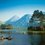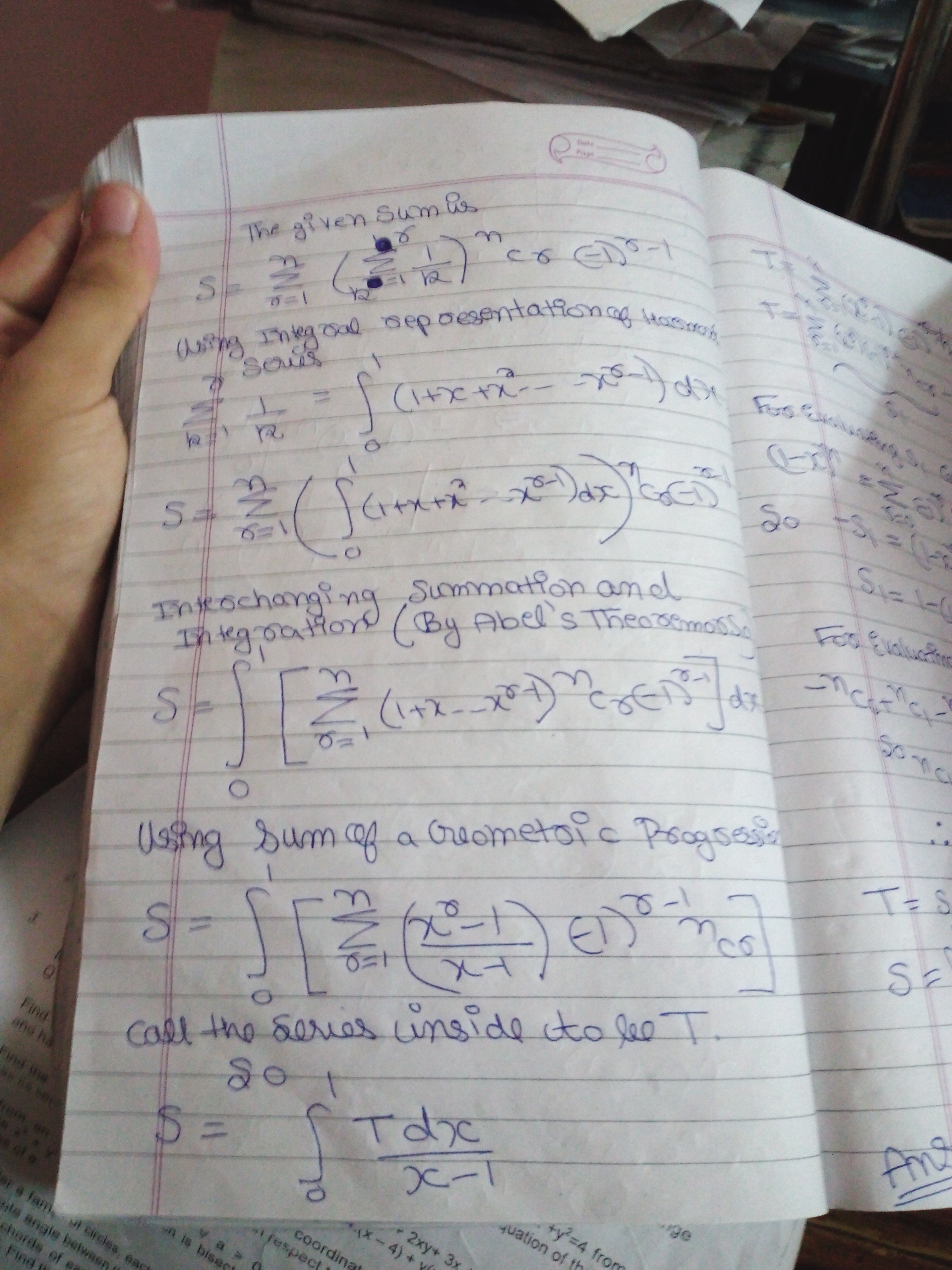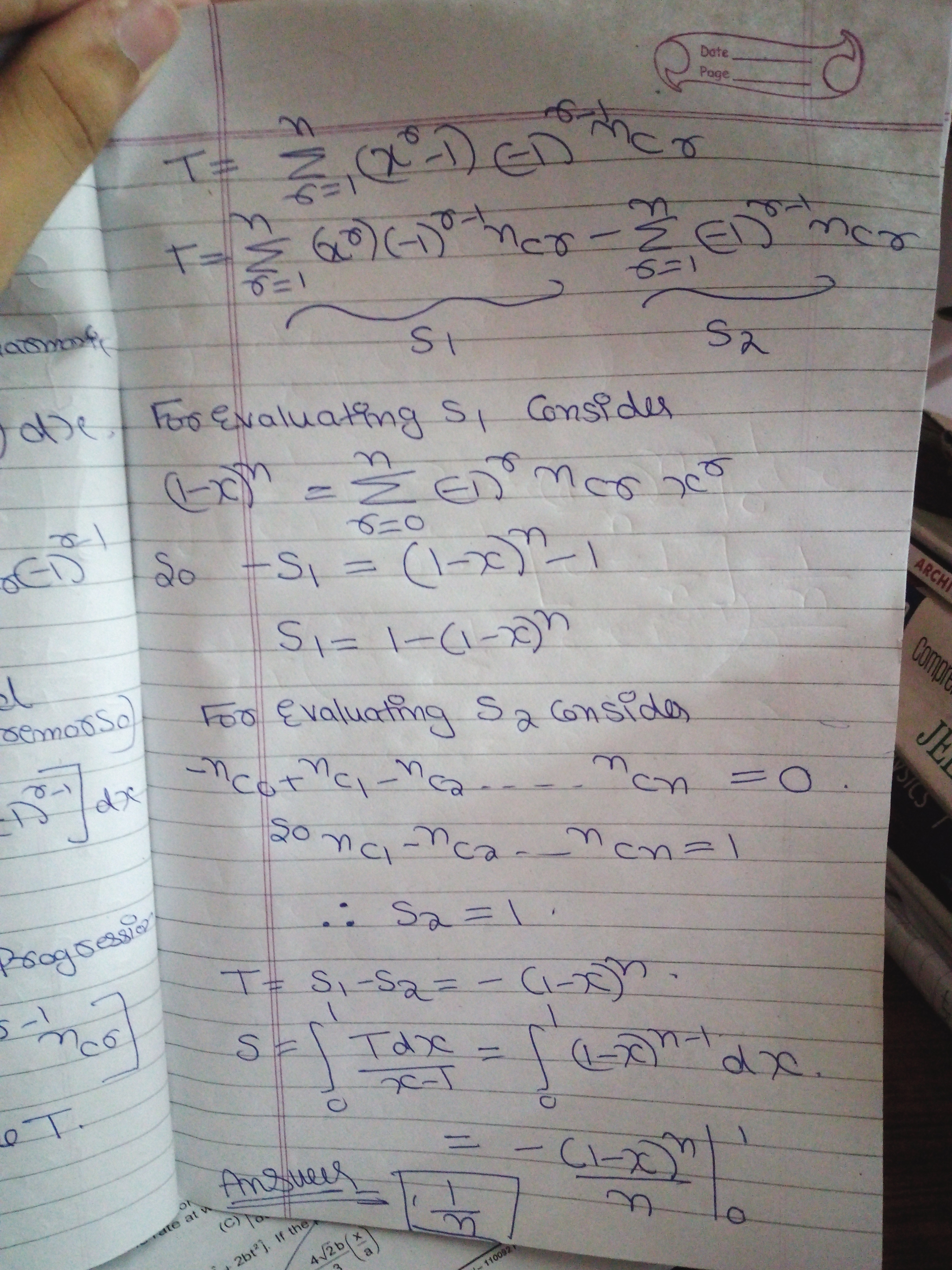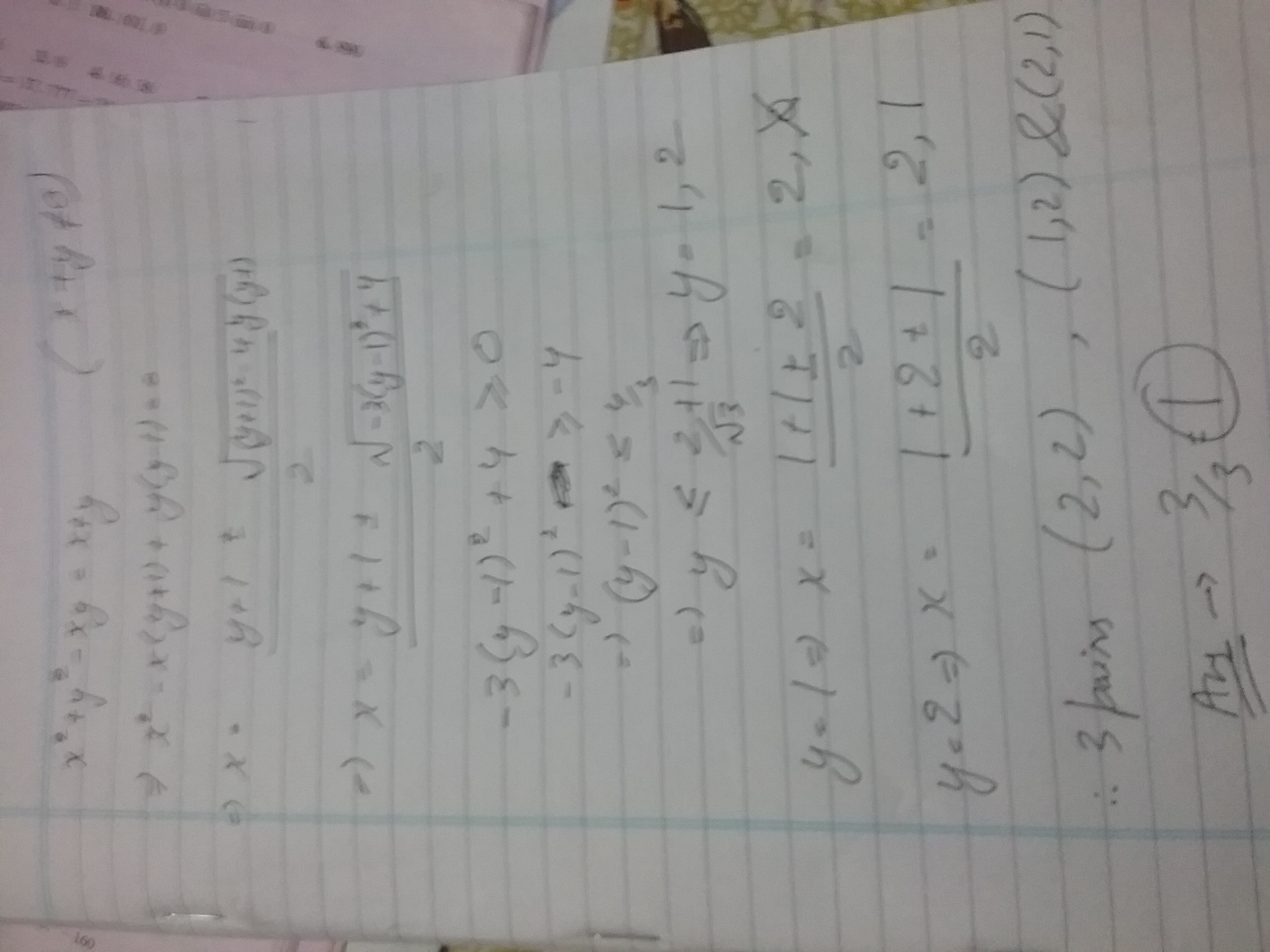# JEE Algebra Contest

TOPICS for week 1+2.

Logarithms, Trigonometry(Ratios and Identies) , Quadratic Equations, Squence and series, Binomial Theorem

Rules

1. I will post the first problem. If someone solves it, he or she can post a solution and then must post a new problem.

2. A solution must be posted below the thread of the problem. Then, the solver must post a new problem as a separate thread.

3. Please make a substantial comment.

4. Make sure you know how to solve your own problem before posting it, in case no one else is able to solve it within 24 hours. Then, you must post the solution and you have the right to post a new problem.

5. If the one who solves the last problem does not post a new problem in 2 hours, the creator of the previous problem has the right to post another problem.

6. It is NOT compulsory to post original problems. But make sure it has not been posted on Brilliant.

7. If a diagram is involved in your problem please make sure it is drawn by a computer program.

8. Format your solution in $\LaTeX$, picture solution will be accepted but only picture will not be, i.e. you can use picture for diagram or something, but not for the complete solution. Also make sure your solution is detailed and make sure to proof all claims.

9. Do not post problems on same topic frequently like 3 continuous inequality problem are not allowed. 2 are sufficient.

10. For those who are on slack Please post in #general that new problem is up along with problem number and the link to contest (https://brilliant.org/discussions/thread/algebra-contest/?sort=new)

11. Handwritten picture solution are accepted only when they are easily understandable!!Note by Rajdeep Dhingra
4 years, 11 months ago

This discussion board is a place to discuss our Daily Challenges and the math and science related to those challenges. Explanations are more than just a solution — they should explain the steps and thinking strategies that you used to obtain the solution. Comments should further the discussion of math and science.

When posting on Brilliant:

• Use the emojis to react to an explanation, whether you're congratulating a job well done , or just really confused .
• Ask specific questions about the challenge or the steps in somebody's explanation. Well-posed questions can add a lot to the discussion, but posting "I don't understand!" doesn't help anyone.
• Try to contribute something new to the discussion, whether it is an extension, generalization or other idea related to the challenge.

MarkdownAppears as
*italics* or _italics_ italics
**bold** or __bold__ bold
- bulleted- list
• bulleted
• list
1. numbered2. list
1. numbered
2. list
Note: you must add a full line of space before and after lists for them to show up correctly
paragraph 1paragraph 2

paragraph 1

paragraph 2

[example link](https://brilliant.org)example link
> This is a quote
This is a quote
    # I indented these lines
# 4 spaces, and now they show
# up as a code block.

print "hello world"
# I indented these lines
# 4 spaces, and now they show
# up as a code block.

print "hello world"
MathAppears as
Remember to wrap math in $$ ... $$ or $ ... $ to ensure proper formatting.
2 \times 3 $2 \times 3$
2^{34} $2^{34}$
a_{i-1} $a_{i-1}$
\frac{2}{3} $\frac{2}{3}$
\sqrt{2} $\sqrt{2}$
\sum_{i=1}^3 $\sum_{i=1}^3$
\sin \theta $\sin \theta$
\boxed{123} $\boxed{123}$

Sort by:

PROBLEM 14

Define f= tan1tan2 + tan2tan3 ..............+tan88tan89

Define g = cot^2(1) -f

define h = log(g)/log(9) where all angles are measured in degrees

Then which of the following statements is true

A) g is prime number

B) g is composite number neither a perfect square nor a perfect cube

C) g is composite number and a perfect square

D) g is composite number and a perfect cube

E) h lies in (1,2)

F) h lies in (2,3)

G) h lies in (3,4)

H) h lies in (0,1)

One or more than one correct type

- 4 years, 11 months ago

$f$ can be written as $\displaystyle \sum_{x=1}^{88} \tan(x) . \tan(x+1)$

As $\displaystyle \tan( (x+1) -x) ) = \dfrac{ \tan(x+1) - \tan(x) }{1 + \tan(x) . \tan(x+1) }$

Using this we get the value of $\displaystyle \tan(x) . \tan(x+1) = \dfrac{ \tan(x+1) - \tan(x)}{\tan(1) } -1$

Summing this we get $f = \cot^2 (1) -89$

So $g = 89$

And $\displaystyle h = \dfrac{\log(89)}{\log(9)}$

- 4 years, 11 months ago

Yes you can post the next question. Its right AF

- 4 years, 11 months ago

Yeah neatly done bro! +1

- 4 years, 11 months ago

- 4 years, 11 months ago

He is gone. Tell complete answer then I will tell. Ik the answer but I cant post

- 4 years, 11 months ago

the answer is B cause cot^2 doesn't give you a perfect square or a perfect cube so it must be B

- 4 years, 11 months ago

Wrong

- 4 years, 11 months ago

Problem 1

If a,b,c $\in$ R - {0}, such that both roots of the equation $ax^2 + bx + c = 0$ lies in (2,3), then both roots of the equation $a{(x+1)}^2 +b(x^2 -1) + c{(x-1)}^2 = 0$ always lies in
A) [1,4]
B)(2,3)
C)[1,3)
D)[1,2)

Type:- ONE OR MORE THAN ONE CORRECT.

- 4 years, 11 months ago

Let the first function be f (x). We know that f (2).f (3)>0 since the roots lie between (2,3) or (4a+2b+c) (9a+4b+c)>0

Let the second be g (x). We know that g (1)=4a and g (2)=9a+4b+c and g (3)=16a+8b+4c=4 (4a+2b+c)

From the above inequality, we figure out that g (3).g (2)>0. So the roots of equation always lie in (2,3) which is included in ABC.

- 4 years, 11 months ago

You may post the next problem.

- 4 years, 11 months ago

For a function g(x),how can one conclude that roots lie between a and b just because g(a)*g(b) > 0 ? Couldn't the graph between a and b be both above/below the x-axis without intersecting and the condition would be still valid but no roots would exist between a and b?

- 2 years, 3 months ago

Problem 5

Consider a polynomial P(x) = $x^2 - 23569x + k$. It has 2 prime number roots. Find the value of k. (Give the answer as sum of digits like 57 = 5+7 = 12 = 1+2 = 3.)

Type:- Integer Type.

- 4 years, 11 months ago

Harsh is right but his answer is wrong

Since 23569 is an odd number,and we know that sum of 2 primes is always even if none of them is 2,therefore two roots of this equation are 2 and 23567.

Thus k = 47134

SOD(k) =19=1+9=10=1+0=1

- 4 years, 11 months ago

Damn I edited my comment.

Who will post the next question?

- 4 years, 11 months ago

Since 23569 is an odd number,and we know that sum of 2 primes is always even if none of them is 2,therefore two roots of this equation are 2 and 23567.

Thus k = 47134

SOD(k) = 1

- 4 years, 11 months ago

Decide who will post the next one

- 4 years, 11 months ago

I have a good problem so can I post because I answered wrong but due to calculation mistake?

- 4 years, 11 months ago

Yeah you can. I have posted so many. I was just writing same solution as you posted and then I got a chance to get this question correct

- 4 years, 11 months ago

PROBLEM 7

$\sin(x)+\sin^{2}(x)=1$ and $a\cos^{12}(x)+b\cos^{10}(x)+c\cos^{8}(x)+d\cos^{6}(x)-2=0$

Find the value of $\frac {a+c+d}{2}$

NOTE a,b,c,d are non zero even integers

Type: Integer type

- 4 years, 11 months ago

SOLUTION 2 :

$sinx = 1 - (sinx)^2 = (cosx)^2$

Now, putting this in the given eq gives

$a (sinx)^6 + b (sinx)^5 + c (sinx)^4 + d (sinx)^3 - 2 = 0$

Now , take cube of equation $sinx + (sinx)^2 = 1$ to get

$(sinx)^6 + 3 (sinx)^5 + 3 (sinx)^4 + (sinx)^3 = 1$

$(sinx)^6 + 3 (sinx)^5 + 3 (sinx)^4 + (sinx)^3 - 1 = 0$

$2 (sinx)^6 + 6 (sinx)^5 + 6 (sinx)^4 + 2 (sinx)^3 - 2 = 0$

- 4 years, 11 months ago

Thats the perfect example of elegance!

- 4 years, 11 months ago

Yeah!! Thanks bro! :)

- 4 years, 11 months ago

Answer to the problem is 5

Firstly Notice the fact that sinx = cos^2(x)

Putting in values we get

asin^6(x) +bsin^5(x)+csin^4(x)+dsin^3(x) = 2 1.....

Now sinx+sin^2(x) = 1

From this we will find values of successive powers of sinx which come to be

sin^2(x) = 1-sinx

sin^3(x) = 2sinx-1

sin^4(x) = 2-3sinx

sin^5(x) = 5sinx-3

sin^6(x) = 5-8sinx

Substituting these values in 1........

And then putting sinx = root 5 -1 /2

In 1..

We will get an expression in terms of a,b,c,d

Now a,b,c,d are integers , RHS is integer therefore irrational term on LHS should be Zero and Integral terms should be equal

Solving you will get

8a-5b+3c-2d = 0

and

18a-11b+7c-4d = 4

Eliminate b from both sides and solving you get a+c+d = 10

Actually the problem was flawed initially.

It took me and @Prince Loomba quite a lot of time to make the problem as it appears now.

Actually According to initial problem statement and answer that prince had only one quadruple was given as the answer. but then i proved that infinitely many quadruples exist. Then prince Told that a,b,c,d are integers . And then after quite a long discussion on slack the final agreement was done that a+c+d has a fixed value not a+b+c+d.

Although are many quadruples exist which satisfy that equation but value of a+c+d will be fixed for each quadruple

- 4 years, 11 months ago

Bro! You can solve this problem in much shorter way , just take cube of $sinx + (sinx)^2 = 1$ ( to get the eq you formed in sin by replacing cos^2 by sin ) .

- 4 years, 11 months ago

PROBLEM 8

Define

K = Tan(27x)-Tan(x)

and

P = sin(x)/cos(3x) +sin(3x)/cos(9x) + sin(9x)/cos(27x)

Assume That x lies in common domain of all the given functions.

Calculate the ratio K/P

And please solve it properly dont just put some value of x to get the ratio .

Type- Integer (0000 to 9999)

- 4 years, 11 months ago

$sinx/cos3x=1/2*(2 sinx cosx/cosx cos 3x)=1/2*(sin2x/cosx cos 3x)=1/2*(tan 3x-tanx)$

Similarly $sin3x/cos9x=1/2*(tan9x-tan3x)$

$sin9x/cos27x=1/2*(tan27x-tan9x)$

Adding these 3 equations, we get $P=1/2*K$ or $K/P=2$

- 4 years, 11 months ago

Yeah right!

- 4 years, 11 months ago

Posted the next one

- 4 years, 11 months ago

PROBLEM 15

Find the value of summation:

$\displaystyle \sum_{r=1}^{k} (-3)^{r-1} \binom{3n}{2r-1}$

where $k = \frac{3n}{2}$ and $n$ is an even positive integer

This is an old JEE question.

- 4 years, 11 months ago

${(1 + \sqrt{x})}^{3n} = 3nC0 + 3nC1 {x}^{0.5} + ........$

${(1 - \sqrt{x})}^{3n} = 3nC0 - 3nC1 {x}^{0.5} + ........$

Subtract the equations and put $x = -3$

You will get

$\frac {{ (1 + \sqrt{3} i )}^{3n} - {( 1 - \sqrt{3} i )}^{3n} }{2\sqrt{3}i } = Required$ $equation$

$\boxed {\frac{{2}^{3n}}{2\sqrt{3}i } ( cos\pi n + i sin\pi n - cos\pi n + sin \pi n ) = 0 }$

- 4 years, 11 months ago

Post problem 16

- 4 years, 11 months ago

If u have one , then post na ! I am not getting what to post

- 4 years, 11 months ago

Ans 0 , RIGHT?

- 4 years, 11 months ago

ya bro!

- 4 years, 11 months ago

Problem 2

Find the minimum value of

|sinx+cosx+tanx+cotx+secx+cosecx|

If the answer is $\theta$, find [$\theta$], where [.] Denotes floor function

TYPE: Integer type

- 4 years, 11 months ago

We make the careful substitution sinx+cosx = t

Converting all in terms of sinx and cosx and writing sinxcosx =(t^2-1)/2

and simplifying i got the expression as

y = t + 2/(t-1)

Just remember we need to find minimum value in (-root 2 , root 2) as range of sinx+cosx is that only

Differentiate this expression to get that function increases from (-infinite,1-root 2) and (1+root 2 , infinite) and decreases in the rest.

So for minimum value of modulus we check that value of t= 1-root 2 and -root 2 the smaller one will be the answer

On solving i got minimum value of modulus = 2root2 -1

And hence box theta = 1

I Dont have much time and good algebra problem so i give the right to @Prince Loomba to post the next

- 4 years, 11 months ago

Problem 3

In a triangle ABC, $-a\leq sin3A+sin3B+sin3C \leq b$. That is this can be minimum -a and maximum b.

Find the value of [a+2b]

Type: Integer type

- 4 years, 11 months ago

Is a=2 and b=2.

- 4 years, 11 months ago

No wrong

- 4 years, 11 months ago

It's more than 24 hours. Post the solution and the next problem.

- 4 years, 11 months ago

Minimum -2 and maximum $3\sqrt 3/2$

- 4 years, 11 months ago

- 4 years, 11 months ago

Let y = sin3A + sin3B + sin3C for sin3A to be non positive

we have 2pi < 3A < 3pi

2pi/3< A <pi

since A + B + C = pi all of sin3A, sin3B, sin3C can’t be negative.

Let us take sin3A = – 1 or A = pi/2

sin3A = – 1, sin3B = – 1 and sin3C = 0 is possible So the minimum value is – 2.

- 4 years, 11 months ago

PROBLEM 4

If $x^{2}+ax+b$ is an integer for every integer x, then

A)a is always an integer but b need not be an integer

B)b is always an integer but a need not be an integer

C)a and b are always integers

D)none of these

Type: Single correct

- 4 years, 11 months ago

C)

- 4 years, 11 months ago

Please post solution and next question

- 4 years, 11 months ago

Put x=0 Then we have b must be an integer Put x=1 Then we have 1+a+b is an innteger Since 1,b both are integers a is also integer

- 4 years, 11 months ago

Ok you can post next question

- 4 years, 11 months ago

Sorry I am a little busy.So I cant.Please u do post

- 4 years, 11 months ago

No I wont. Its your turn.

- 4 years, 11 months ago

Should we also start a mechanics contest again?

- 4 years, 11 months ago

As soon as this contest or any one of the other ends we will immediately start up with mech .... btw I also wanted to start up with Analytical and regular NT contest ,,, ;)

- 4 years, 11 months ago

After mechanics, we will start NT :)

- 4 years, 11 months ago

Bro try Problem 7

- 4 years, 11 months ago

3 contests are running now. Later. Now: geometry,algebra,jee algebra

- 4 years, 11 months ago

Hey guys @Harsh Shrivastava @Chinmay Sangawadekar @Rajdeep Dhingra the problem is corrected now it was a bit flawed initially

- 4 years, 11 months ago

Problem 9

Consider a rational function of the form of

$\frac {a_{0}x^{0}+a_{1}x^{1}+.....+a_{m}x^{m}}{b_{0}x^{0}+b_{1}x^{1}+.....+b_{n}x^{n}}$

As $x$ tends to $\infty$, the function tends to $\infty$.

It is given that $0\leq m,n \leq 10$

If the number of ordered pairs $(m,n)$ that satisfy the given conditions is $c$,

Find $SOD (c)$

Note $SOD$ denotes repeated sum of digits for example

$SOD(189)=SOD (1+8+9)=SOD (18)=SOD (1+8)=SOD (9)=9$.

Type: Integer type

- 4 years, 11 months ago

The given function will tend to $\infty$ when m>n . So we have to find ordered pairs (m,n) in $[0,10]$ where m>n which is equal to $\binom{11}{2} = 55$.

SOD(55)=SOD(10)=1.

- 4 years, 11 months ago

Right. Are you posting next one or shall I?

- 4 years, 11 months ago

I don't have any good question to post at the moment so you may post the next one.

- 4 years, 11 months ago

Problem 10

Lets define a function named prince (x,n) such that prince (x,n)=[x]+[x/n]+...+[x/$n^{\infty}$]

Find the SOD (prince (10000,50)) without calculating each floor function value

Type: Integer type

- 4 years, 11 months ago

- 4 years, 11 months ago

No and SOD stands for sum of digits, refer problem 9

- 4 years, 11 months ago

$\lfloor \frac{a}{b}\rfloor = 0$ if b>a.

Therefore, the sum is effective only till $i$ where $i < 3$.

Therefore answer = SOD(10000+200+4) = SOD(10204) = 7.

- 4 years, 11 months ago

Problem 12 IF $a$ and $b$ are the roots of equation $x+1=c x (1-cx)$ and $c_{1},c_{2}$ be two values of $c$, determined from the equation $\frac {a}{b}+\frac {b}{a}=\pi -2$.

If $\frac {c_{1}^{2}}{c_{2}^{2}}+\frac {c_{2}^{2}}{c_{1}^{2}}+2=k (\frac {\pi+1}{\pi-1})^{2}$, find the value of $k$.

Type: Integer type

- 4 years, 11 months ago

K = 4 ; I will edit sol after coming back from my class

- 4 years, 11 months ago

4 is right. Post solution and then the next question after coming.

- 4 years, 11 months ago

$c^{2}x^{2}-x(c-1)+1=0$

$\dfrac{a}{b}+\dfrac{b}{a} = \dfrac{a^{2}+b^{2}}{ab} = (c-1)^{2}-2$

$(c-1)^{2}-2=\pi-2$

$c^{2}-2c+1-\pi = 0$

$\dfrac{c_{1}^{2}}{c_{2}^{2}}+\dfrac{c_{2}^{2}}{c_{1}^{2}} +2 = \dfrac{(c_{1}^{2}+c_{2}^{2})^{2}}{c_1^{2}c_2^{2}}$

$c_{1}^{2}+c_{2}^{2} = 4 - 2(1-\pi) = 2\pi + 2$

$\dfrac{c_{1}^{2}}{c_{2}^{2}}+\dfrac{c_{2}^{2}}{c_{1}^{2}} +2 = \dfrac{(2\pi+2)^{2}}{(\pi-1)^{2}}$

$\boxed{k}=4$

Someone else may post the next question. @Prince Loomba @Aniket Sanghi

- 4 years, 11 months ago

Problem 13

Let$-1\leq p \leq 1$

Show that the equation $4x^{3}-3x-p=0$ has $1$ unique root in the interval $[1/2,1]$ and identify it. This is JEE problem with exact wordings.

- 4 years, 11 months ago

is the root 1

- 4 years, 11 months ago

No it is in terms of p

- 4 years, 11 months ago

oh then the answer must be p+1

- 4 years, 11 months ago

No and p+1 is out of the given range

- 4 years, 11 months ago

Looking at the range you should definitely think of sine and cosine functions as there range is also the same.

Then recall the trigonometric identity cos(3x) = 4cos^3(x)-3cos(x)

So we carefully substitute x = cos(y)

Now problem is over!.

The root will cos[arccos(p)/3]

- 4 years, 11 months ago

- 4 years, 11 months ago

- 4 years, 11 months ago

problem 16

If x={$3^{2n}/8$} where {} denotes fractional part

Find value of $sec^{-1}8x$

- 4 years, 11 months ago

$x = \dfrac{3^{2n}}{8}= \dfrac{9^{n}}{8} = \dfrac{(8+1)^{n}}{8} = \dfrac{\binom{n}{0}8^{n}+\binom{n}{1}8^{n-1}+....+ \binom{n}{n-1}8+ \binom{n}{n}}{8} = {\dfrac{1}{8}}=\dfrac{1}{8}$

$sec^{-1}(1) = 0$

There are supposed to be { } around every fraction which i was not able to add.

- 4 years, 11 months ago

Right post next

- 4 years, 11 months ago

problem 17

Find the value of $\displaystyle \sum_{r=0}^{n}{\frac {^{n}C_{r}}{r+1}}$

- 4 years, 11 months ago

we know that

$\displaystyle (1+x)^n = \sum_{r=0}^{n} \binom{n}{r}$

Simply integrating it from 0 to 1 gives the required result

So answer is $\displaystyle \int_{0}^{1} (1+x)^n dx = \dfrac{2^{n+1} -1}{n+1}$

- 4 years, 11 months ago

Post next question

- 4 years, 11 months ago

Bro! It is $\boxed{\frac{ {2}^{n+1} - 1 }{ n + 1}}$

- 4 years, 11 months ago

Thanks for that I have edited it

- 4 years, 11 months ago

I saw the method and not the answer and thats why I thought it to be right!

- 4 years, 11 months ago

PROBLEM 18

Evaluate

$\displaystyle \sum_{r=1}^{n-1} \left( \sum_{k=1}^r \dfrac 1k \right) \times (-1)^{r-1} \binom{n}{r}$

- 4 years, 11 months ago

I Am attaching photos of my solution- 4 years, 11 months ago

Are you sure that this series converges?

- 4 years, 11 months ago

Sorry for inconvenience, I have edited the question

- 4 years, 11 months ago

Bro initially sigma should be from 1 to n not n-1 !!

- 4 years, 11 months ago

I dont have any question so you can post next!

- 4 years, 11 months ago

Problem 19

How many numbers between $1$ and $10000$ ,both inclusive contain different digits when represented in the scientific notation?

Example: $3450=3.45×10^{3}$ is right and $4010=4.01×10^{3}$ is right but $4001=4.001×10^{3}$ is wrong as it contain 2 zeroes.

- 4 years, 11 months ago

1st make it clear , is number 4000 right? (Means can it be counted ?)

- 4 years, 10 months ago

Yes 4000 is right 4.0×10^3. I have given such example for 4010 bro

- 4 years, 10 months ago

Problem 20

A=(1/1) arccot(1/1)+(1/2) arccot (1/2)+(1/3) arccot (1/3) and B= (1) arccot (1)+(2)arccot(2)+(3)arccot(3)

Find the value of |B-A|

- 4 years, 10 months ago

Allen test paper question.

- 4 years, 10 months ago

Bro I know haha

- 4 years, 10 months ago

Ik that Bro

- 4 years, 10 months ago

Answer has to be in which form ? @Prince Loomba

- 4 years, 10 months ago

(a/b)×pi+(c /d)arccot3

- 4 years, 10 months ago

Post next problem anybody.

- 4 years, 10 months ago

Problem 6

Let number of pairs of positive integers that satisfy the equation $x^3 +y^3 = (x+y)^2$ be $n$.

Find the value of $n/3$.

Type-: Integer Type.

- 4 years, 11 months ago

See the first line of the note

- 4 years, 11 months ago

Post other. Its out of the topics list

- 4 years, 11 months ago

Well I have read the note and I very well know what to post.

The problem is alright.

It is within topic.

- 4 years, 11 months ago

Its infinite

- 4 years, 11 months ago

If I tell the topic. the problem will become very easy

- 4 years, 11 months ago

Its infinite. x=0 y=any nonnegative integer

- 4 years, 11 months ago

Sorry correction done.

- 4 years, 11 months ago

Please see typo has been corrected.

- 4 years, 11 months ago

Now its 0

- 4 years, 11 months ago

Now its 0, 3xy (x+y)=0 implies x=0 or y=0 or x=-y. Any of the case is not possible

- 4 years, 11 months ago

You committed a common mistake.

- 4 years, 11 months ago

Then tell me one of the pairs so that I get my answer is incorrect

- 4 years, 11 months ago

(2,2).

- 4 years, 11 months ago

2^3+2^3=16 and 4^3=64. Wrong

- 4 years, 11 months ago

Bro I am really sorry.

I have mistyped the question.

I have edited the question one last time.

Sorry.

- 4 years, 11 months ago

Try your luck again. I am right

- 4 years, 11 months ago

I have edited the question.

- 4 years, 11 months ago

Aftr the calculations, answer is 1 and the 3 pairs are 2,2 , 1,2 , 2,1

- 4 years, 11 months ago- 4 years, 11 months ago

Correct post the next question.

- 4 years, 11 months ago

Problem 11

Given that the maximum value of $\displaystyle \prod_{i=1}^{n}(1+\sin ^2 x_{i})(1 + \cos ^2 x_{i} )$ is the minimum value of $\dfrac{9^{10}}{2}y^{10} + \dfrac{2}{4^{10}y^{10}}$.

Find the value of n.

Type : Integer Type.

- 4 years, 11 months ago

The product is max when all terms equal 3/2. Or we can write it as $(3/2)^{2n}$

The min value of the second as calculate by AM-GM is $(3/2)^{10}$.

From these 2 we can conclude $n=5$

- 4 years, 11 months ago

- 4 years, 11 months ago

Whaat is wrong. Differentiate. 1 more thing, the min value is 2 times of that I have written. This impies that n is not integer

- 4 years, 11 months ago

Justify your assumption why max occurs when all terms are equal?

- 4 years, 11 months ago

Actually it's just observation that a product is maximum when all terms are as close as possible, differentiation can also be used!

- 2 years, 3 months ago